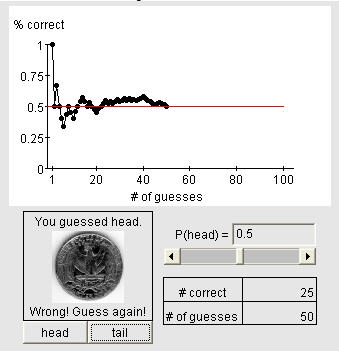# Psychic test

This material is replicated on a number of sites as part of the SERC Pedagogic Service Project

## Summary

This activity illustrates the convergence of long run relative frequency to the true probability. The psychic ability of a student from the class is studied using an applet. The student is asked to repeatedly guess the outcome of a virtual coin toss. The instructor enters the student's guesses and the applet plots the percentage of correct answers versus the number of attempts. With the applet, many guesses can be entered very quickly. If the student is truly a psychic, the percentage correct will converge to a value above 0.5.

## Learning GoalsThe goal of the activity is to help students understand the idea of relative frequency converging to true probability as the number of trials increases. Secondary to that, the activity starts the motivation for hypothesis testing as students are asked to determine a criterion for psychic ability. The applet also reinforces the idea of sampling variability and how it decreases with sample size.

## Context for Use

This activity is designed to be used after a discussion of elementary probability. Specifically, the activity should be used after a statement relating long run relative frequency to true probbility (N(A)/N converges to P(A)). This activity will work in any lecture or lab regardless of the size as long as it is equipped with a computer and internet connection. The activity should take less than 10 minutes.

## Description and Teaching Materials

The applet is located at http://www.stat.tamu.edu/~west/applets/psychictest.html.

## Teaching Notes and Tips

The instructor should poll the class for potential psychic ability. After selecting an eager potential psychic, the instructor should ask the class what the criterion for being a psychic is. This discussion should eventually lead to the idea of P(predicting the future correctly) much higher than average.

The instructor then introduces the coin tossing applet. The instructor should then return to the original discussion and ask what psychic ability would mean in the context of the applet. The discussion should focus around the idea of P(correctly guessng a toss) > 0.5, the probability associated with an average person randomly guessing the outcome. This value is indicated with a red line in the applet.

The instructor should then ask the class how to use the applet to gauge the student's psychic ability. This will lead to having the student make a large number of guesses in order to estimate the probability of a correct guess. The instructor should have the student make at least 50 guesses with the instructor entering each response for the student by clicking the head and tail buttons. Using the applet allows the guesses to be entered very quickly. Most of the time 50 trials provides a reasonable pattern of convergence.

One can always keep the final percentage/number of guesses and do a formal hypothesis test for psychic ability later in the semester.

## Assessment

Ask follow up questions such as "if the student is an antipsychic (unlucky), to what value will the percent correct converge?" Answers to this question provide feedback as to whether the students understand the concept. On subsequent exams, one can ask questions in another context to see if students get the idea. For example, one might ask the multiple choice question below:

A quality control engineer believes that the probability of producing a defective item from a manufacturing process is 0.1. Which of the following is true if the engineer's statement is correct?

• The number of defects observed in a large number of sample items produced from the process will converge to 0.1.
• The proportion of defective items in a large number of sample items produced from the process will converge to 0.1.
• The number of defects observed in a large number of sample items produced from the process will converge to 0.5.
• The proportion of defective items in a large number of sample items produced from the process will converge to 0.5.
• 1 out of 10 items produced from the process will be defective.

## References and Resources

http://www.stat.tamu.edu/~west/applets/psychictest.html